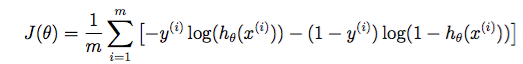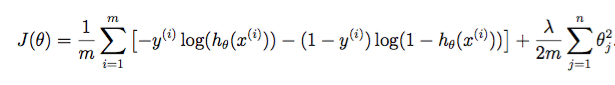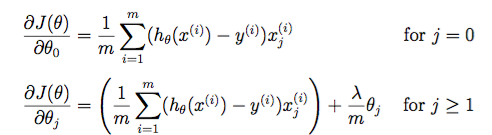# PuGong

20 Feb 2016

## Machine Learning Notes

### Neural Networks: Representation

if there are too many features, the linear regression will be two large to be calculated. For example: suppose 100*100 pixel images (grayscale, not RGB), for term(XiXj) the features will be up to (5 * 10^7)

#### Cost function

• unregularized logistic regressionwhere• regularized logistic regressionwhere• neural network### Neural Networks: Learning

#### Backprogagation Algorithm#### Reference:

Course Home in Coursera

Course Homepage in Stanford

Course Materials